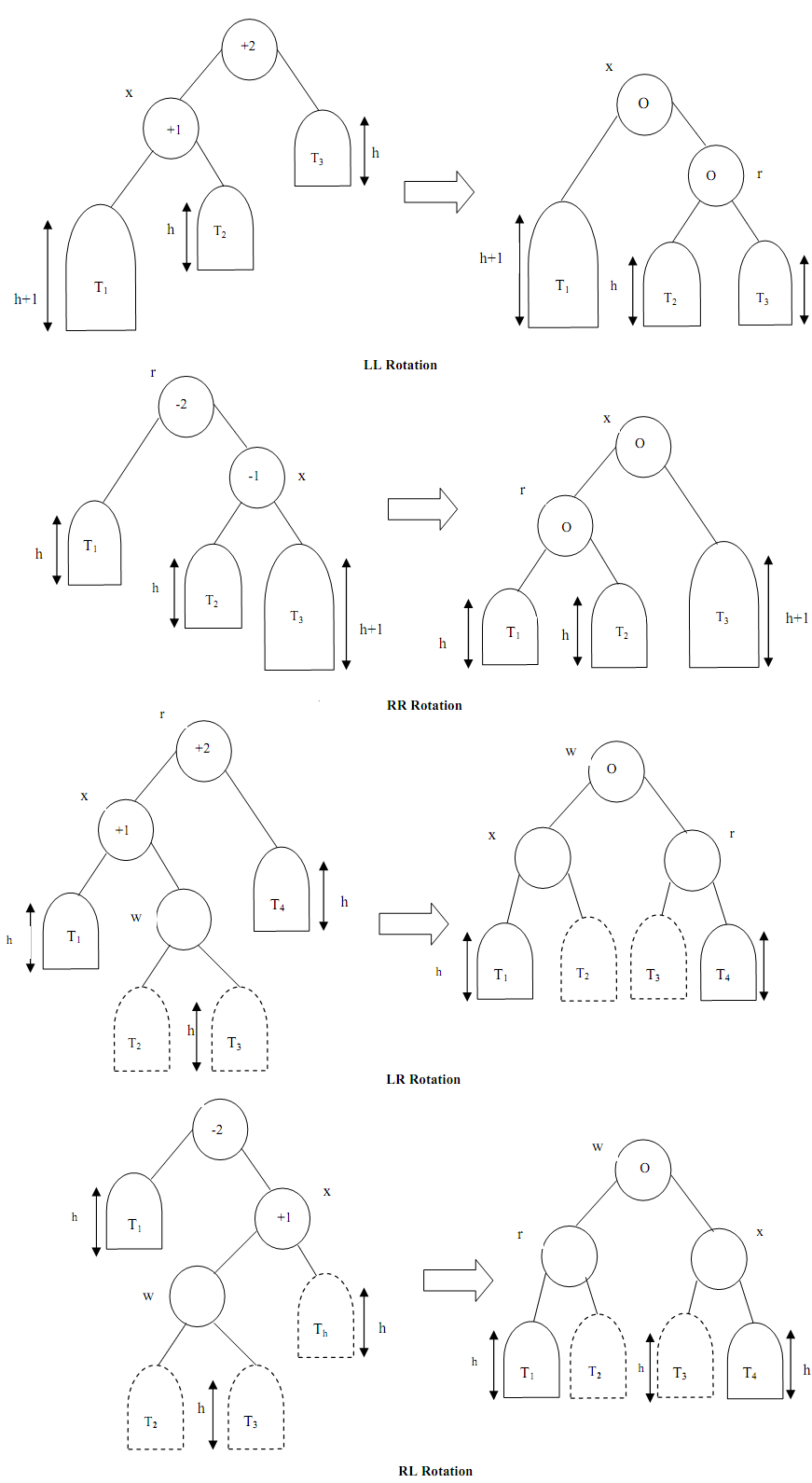## Avl tree rotations, Data Structure & Algorithms

Assignment Help:

AVL trees and the nodes it contains must meet strict balance requirements to maintain O(log n) search time. These balance restrictions are kept maintained via various rotation functions.

The four possible rotations which can be performed on an unbalanced AVL tree are given below. The before & after status of an AVL tree needing the rotation are illustrated#### If, 1. Start 2. Get h 3. If h T=288.15+(h*-0.0065) 4. else if h T=2...

1. Start 2. Get h 3. If h T=288.15+(h*-0.0065) 4. else if h T=216.65 5. else if h T=216.65+(h*0.001) 6. else if h T=228.65+(h*0.0028) 7. else if h T=270.65 8.

#### Multiple stacks, how multiple stacks can be implemented using one dimension...

how multiple stacks can be implemented using one dimensional array

#### Mapping constain, one to many one to one many to many many to one

one to many one to one many to many many to one

#### Traversing a binary search tree, Binary Search Tree let three types of trav...

Binary Search Tree let three types of traversals by its nodes. They are: Pre Order Traversal In Order Traversal Post Order Traversal In Pre Order Traversal, we ca

#### Aa-trees, Red-Black trees have introduced a new property in the binary sear...

Red-Black trees have introduced a new property in the binary search tree that means an extra property of color (red, black). However, as these trees grow, in their operations such

#### Abstract Data Types, A useful tool which is used for specifying the logical...

A useful tool which is used for specifying the logical properties of a data type is called the abstract data type or ADT. The term "abstract data type" refers to the fundamental ma

#### Time complexity, how to learn about time complexity of a particular algorit...

how to learn about time complexity of a particular algorithm

#### Introduction, what are avl trees

what are avl trees

#### Dijkstras algorithm, Djikstra's algorithm (named after it is discovered by ...

Djikstra's algorithm (named after it is discovered by Dutch computer scientist E.W. Dijkstra) resolves the problem of finding the shortest path through a point in a graph (the sour

#### Depth of complete binary tree, What will be depth do , of complete binary t...

What will be depth do , of complete binary tree of n nodes, where nodes are labelled from 1 to n with root as node and last leaf node as node n